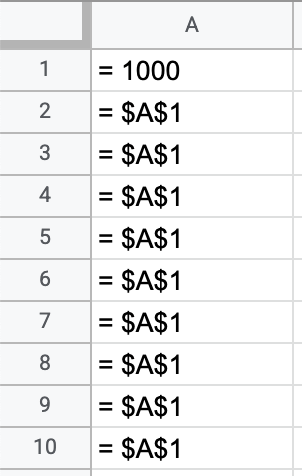# Optimize your data references to improve Sheets performance

Reference your data in Sheets to improve performance when you work with large data sets. Follow these suggestions to improve:

• Computation speed
• Stability
• CPU usage

## Reference data on the same Sheet when possible

Reference your data on the same spreadsheet you work on. This is faster than Import functions, such as:

• IMPORTRANGE
• IMPORTDATA
• IMPORTXML
• IMPORTHTML

If you use IMPORTRANGE() to pull data from another spreadsheet, the Import function that references data between the spreadsheets still goes through the internet, even if you:

• Own the spreadsheet.
• Open it in the same browser.
• Have it located in the same drive.

This requires a round trip to request and fetch data. You may experience delays and intermittent connections that slow load speed.

If you manually move data from another spreadsheet to your own spreadsheet and reference your data from it (including different tabs from the same spreadsheet), the process to fetch data happens locally, not through the internet. This will save time, but only applies when you work on relatively static and deterministic data that isn’t subject to change, for example, inventory history or historical data.

To manually move data, copy existing data from another spreadsheet to your spreadsheet:

1. On the bottom, next to the sheet's name, click Down arrow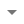Copy toExisting spread sheet.
2. Select a spreadsheet to copy this sheet into.

Tip: If you can't find the spreadsheet to copy to in the Drive view, paste the spreadsheet's web address into the bottom bar.

## Use closed range instead of open range references

An open range spreadsheet means the range starts and ends without indicating a specific row or column. Example: A:B means the range that includes all cells in columns A and B.

A closed range reference refers to the range that starts and ends with a specific row or column.
Example: A1:B6, A1:C100.

Open range: A:B

Closed range: A1:B6

Example: Imagine you are calculating the sum of column A, but only the first 10 out of 10,000 rows contain a value.

• If you use an open range reference in a SUM function, SUM(A:A), your computer reads all 10,000 rows, even though there are empty cells in the open range reference. Google Sheets goes through each of them to make sure they aren’t empty.
• If you use the close reference, SUM(A1:A10), your computer only reads rows A1 to A10 and Sheets computes faster.

## Reference your volatile function efficiently

TODAY(), RAND(), RANDBETWEEN(), and NOW() are volatile functions because they change and refresh frequently to keep up to date. These functions aren’t static in nature. For example, TODAY() refreshes every day.

Example: Each row of column B reads data from each row of column A. That means column A refreshes all cells when column B calculates the results.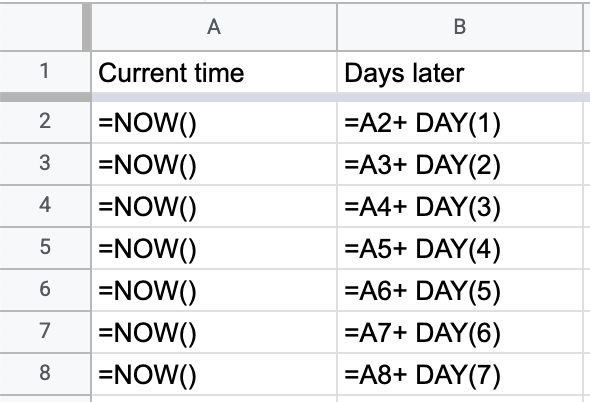When you reference volatile functions that give you the same result, such as NOW() and TODAY(), make sure to use absolute reference so you only reference one time. In this case, column B calculates results depending on one cell, A2. When you remove the unnecessary dependency, your Sheets run faster.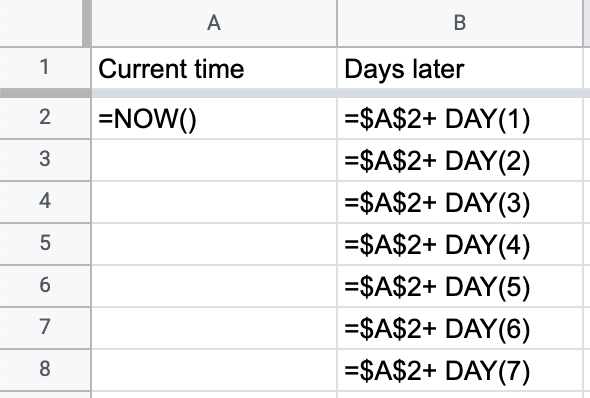## Avoid long reference chains when possible

Reference chains slow down your sheets. For example, in the following case, A2 reads A1 data; A3 reads A2 data; and so on. Because the data is chained together, to calculate the value in A10, Google Sheets waits for all the previous values (A1 to A9) to calculate before it returns the value in A10.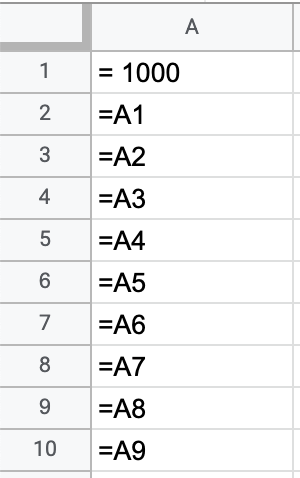To avoid chained calculations when you fill the cells, use absolute references. In this case, A2 reads data from A1, A3 reads data from A1, and so on. Since A1 is calculated and available, A2 to A10 directly fetches A1’s value. The result is identical but the calculation is faster.